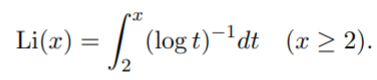# Logarithmic Integral Function: Definition

The logarithmic integral function Li(x) (or just the “logarithmic integral”) is a locally summable function on the real line.

This special function is used in physics and number theory, most notably in the prime number theorem. One of the earliest references to the function was in 1986, when Jacques Hadamard and de la Vallée Poussin independently proved the prime number theorem, showing that if π(x) is the number of primes up to x, then for any positive constant ܿc, π(x) = Li(x) + O(xe-c√(ln x)).

The formula for the logarithmic integral function for positive values of x isSome versions of the integral have a lower bound of integration of 0 (called the American version) instead of 2 (the European version). The two integrals are related by the formula (x) = Li(x) – Li(2) = Li(x) – 1.045163780 . The European version avoids the problem of a singularity at t = 1; Another way to avoid this problem is to use a set of integrals (where PV is the integral’s Cauchy principal value) :The integrals of the functions (log t)−1 and e−t2 define the logarithmic integral. These cannot be integrated in terms of elementary functions .

## Applications of the Logarithmic Integral Function

The logarithmic integral function is used primarily in physics and number theory.

In number theory, the function makes an appearance in the prime number theorem. The theorem states that as x tends to infinity, the number of primes less than x can be approximated byWhere li(x) is the logarithmic integral .

## References

 Pardo, J. (2012). Cryptography and Cryptanalysis: Primality, Factoring and Discrete Logarithms. Springer.
 Fisher, B. & Al-Sihery, F. (2016). On the Logarithmic Integral and the Convolution. Mathematica Moravica.
 Hildebrand, A. (2015). Short Course on Asymptotics. Mathematics Summer REU Programs. University of Illinois. Retrieved April 5, 2021 from: https://faculty.math.illinois.edu/~hildebr/lecture-notes/asymptotics.pdf